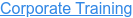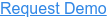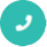#### Digital Marketing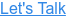# Python Pandas Interview Questions and Answers

by sonia, on May 19, 2017 3:56:27 PMAre you trying for a Python job? Here are the top frequently asked interview questions and answers to step-on the python interview. Dive into these Python interview questions and answers and see just how well-versed you are in this Python language.

## Q1. What is Pandas/Python Pandas?

Ans: Pandas is a Python package providing fast, flexible, and expressive data structures designed to make working with “relational” or “labeled” data both easy and intuitive. It aims to be the fundamental high-level building block for doing practical, real world data analysis in Python.

## Q2. What is Python pandas used for?

Ans: pandas is a software library written for the Python programming language for data manipulation and analysis. In particular, it offers data structures and operations for manipulating numerical tables and time series. pandas is free software released under the three-clause BSD license.

## Q3. What is a pandas DataFrame?

Ans: DataFrame is a 2-dimensional labeled data structure with columns of potentially different types. You can think of it like a spreadsheet or SQL table, or a dict of Series objects. It is generally the most commonly used pandas object.

## Q4. What is NP Python?

Ans: NumPy (pronounced /ˈnʌmpaɪ/ (NUM-py) or sometimes /ˈnʌmpi/ (NUM-pee)) is an extension to the Python programming language, adding support for large, multi-dimensional arrays and matrices, along with a large library of high-level mathematical functions to operate on these arrays.

## Q5. What is Matplotlib?

Ans: matplotlib is a plotting library for the Python programming language and its numerical mathematics extension NumPy. It provides an object-oriented API for embedding plots into applications using general-purpose GUI toolkits like wxPython, Qt, or GTK+.## Q6. What can I import from NumPy?

Ans: numpy is the top package name, and doing import numpy doesn'timport submodule numpy.f2py . ... The link is established when you do import numpy.f2py. In your above code: import numpy as np # np is an alias pointing tonumpy, but at this point numpy is not linked to numpy.f2py import numpy.

## Q7. What is Scipy?

Ans: SciPy (pronounced "Sigh Pie") is open-source software for mathematics, science, and engineering. It is also the name of a very popular conference on scientific programming with Python. The SciPy library depends on NumPy, which provides convenient and fast N-dimensional array manipulation.

## Q8. What is plot ly?

Ans: Plotly, also known by its URL, Plot.ly, is an online analytics and data visualization tool, headquartered in Montreal, Quebec.

## Q9. What is PIP for Python?

Ans: pip is a package management system used to install and manage software packages written in Python. Many packages can be found in the Python Package Index (PyPI). Python 2.7.9 and later (on the python2 series), and Python 3.4 and later include pip (pip3 for Python 3) by default.

## Q10. What is Sympy?

Ans: SymPy is a Python library for symbolic mathematics. It aims to become a full-featured computer algebra system (CAS) while keeping the code as simple as possible in order to be comprehensible and easily extensible.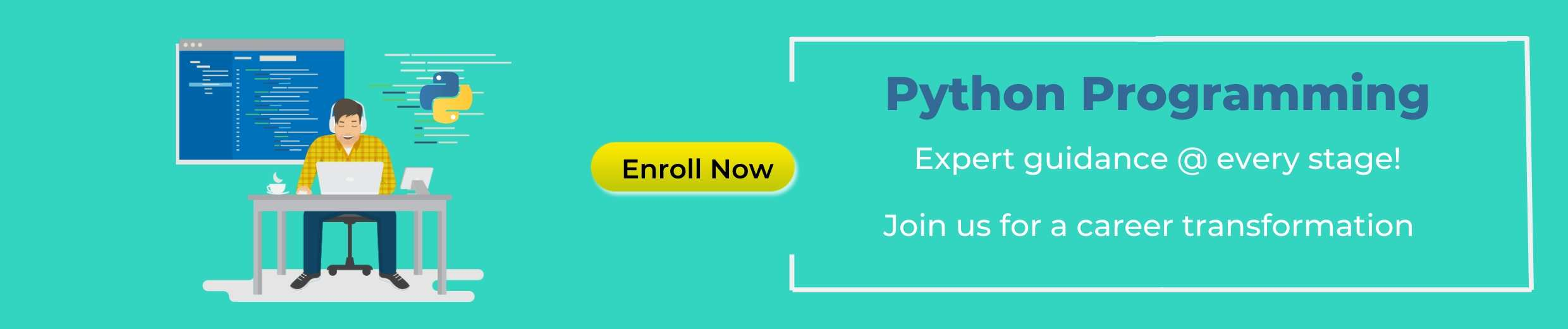Related Interview Questions...